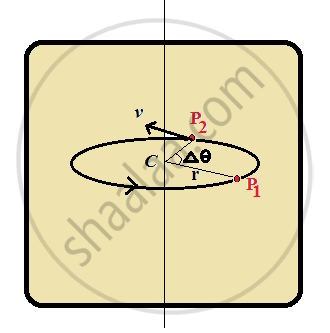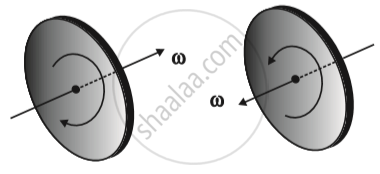# Angular Velocity and Its Relation with Linear Velocity

#### description

• Relation Between Linear Velocity and Angular Velocity

#### notes

Angular velocity & its relation with linear velocity

Every particle of a rotating body moves in a circle. Angular displacement of a given particle about its centre in unit time is defined as angular velocity.• Average angular velocity = ΔΘ/Δt

• Instantaneous angular velocity, ω = dΘ / dt

• v = wr, where v – linear velocity of particle moving in a circle of radius r

• All parts of a moving body have the same angular velocity in pure rotation motion.

• Angular velocity, ω, is a vector quantity

• If you curl your fingers of right hand in the sense of rotation, thumb will give direction of angular velocity.• V = ω x r

• Angular Acceleration is given by rate of change of angular velocity with respect to time.

• α = dω / dt

If you would like to contribute notes or other learning material, please submit them using the button below.

#### Video Tutorials

We have provided more than 1 series of video tutorials for some topics to help you get a better understanding of the topic.

Series 1

Series 2

### Shaalaa.com

Relation between linear velocity and angular velocity [00:08:37]
S
0%